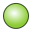# KU Card

This card creates a mesh of surface triangles in the shape of a spherical section.

On the Construct tab, in the Surfaces group, click theSphere (KU) icon.

## Parameters:

S1
The centre of the sphere.
S2
A point that indicates the $\vartheta =0°$ direction in a spherical coordinate system. The distance between S1 and S2 is the radius of the sphere.
S3
A point that indicates the $\vartheta =90°$ , $\phi =0°$ direction in a spherical coordinate system. The distance S1–S3 must be equal to the distance S1–S2.
Normal direction
The triangles can be created so that the normal vectors point Outward (away from the centre of the sphere) or Inward (towards it).
Begin angle ${\vartheta }_{a}$ (degrees)
The start angle ${\vartheta }_{a}$ in degrees of the spherical segment.
Begin angle ${\phi }_{a}$ (degrees)
The start angle ${\phi }_{a}$ in degrees of the spherical segment.
End angle ${\vartheta }_{e}$ (degrees)
The end angle ${\vartheta }_{e}$ in degrees of the spherical segment.
End angle ${\phi }_{e}$ (degrees)
The end angle ${\phi }_{e}$ in degrees of the spherical segment.
Maximum edge length (bottom)
The maximum length of the triangles along the curved edges in m (is scaled by the SF card). If this parameter is left empty, the value specified with the IP card is used.

A complete sphere may be created with ${\vartheta }_{a}={\phi }_{a}=0$ , ${\vartheta }_{e}=180°$ and ${\phi }_{e}=360°$ .

## An example of KU card usage:

The spherical segment is generated using the KU card.